# KSEEB SSLC Class 10 Maths Solutions Chapter 2 Triangles Ex 2.2

KSEEB SSLC Class 10 Maths Solutions Chapter 2 Triangle Ex 2.2 are part of KSEEB SSLC Class 10 Maths Solutions. Here we have given Karnataka SSLC Class 10 Maths Solutions Chapter 2 Triangle Exercise 2.2.

## Karnataka SSLC Class 10 Maths Solutions Chapter 2 Triangle Exercise 2.2

Question 1.
In the following fig. (i) and (ii). DE || BC.
Find EC in (i) and AD in (ii).Solution:
(i) In ∆ABC, DE || BC, EC =?
$$\quad \frac{\mathrm{AD}}{\mathrm{BD}}=\frac{\mathrm{AE}}{\mathrm{EC}}$$
$$\frac{1.5}{3}=\frac{1}{\mathrm{EC}}$$
1.5 EC = 1 × 3
$$\mathrm{EC}=\frac{3}{1.5}$$
$$E C=\frac{30}{15}$$
∴ EC = 2 cm

(i) In ∆ABC, DE || BC, AD =?
$$\frac{\mathrm{AD}}{\mathrm{BD}}=\frac{\mathrm{AE}}{\mathrm{EC}}$$
$$\frac{\mathrm{AD}}{7.2}=\frac{1.8}{5.4}$$
$$\quad A D=\frac{1.8}{5.4} \times \frac{7.2}{1}$$
$$=\frac{1.8}{54} \times \frac{72}{1}$$
= 0.6 × 4

Question 2.
E and F are points on the sides PQ and PR respectively of a PQR For each of the following cases, state whether EF || QR:
(i) PE = 3.9cm. EQ = 3 cm
PF=3.6cm. FR = 2.4 cm.
(ii) PE = 4 cm. QE = 4.5 cm.
PF = 8 cm. RF = 9 cm.
(iii) PQ = 1.28 cm. PR = 2.56 cm.
PE = 0.18 cm. PF = 0.36 cm.
Solution:In ∆PQR, if EF || QR then,
$$\frac{\mathrm{PE}}{\mathrm{QR}}=\frac{\mathrm{PF}}{\mathrm{FR}}$$
$$\frac{3.9}{3}=\frac{3.6}{2.4}$$
1.3 ≠ 1.5
Sides are not dividing In ratio.
∴ EF is not parallel to QR.

(ii)In ∆PQR, if EF || QR then
$$\frac{\mathrm{PE}}{\mathrm{QR}}=\frac{\mathrm{PF}}{\mathrm{FR}}$$
$$\frac{4}{4.5}=\frac{8}{9}$$
$$\frac{8}{9}=\frac{8}{9}$$
Here sides are dividing in ratio.
∴ EF ||QR

(iii)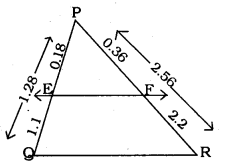PE + EQ = PQ
0.18 + EQ = 1.28
∴ EQ=1.28 – 0.18
EQ = 1.1 cm.
Similarly. PF + FR = PR
0.36 + FR = 2.56
FR = 2.56 – 0.36
FR = 2.2cm.
In ∆PQR, if EF || QR then
$$\frac{\mathrm{PE}}{\mathrm{QE}}=\frac{\mathrm{PF}}{\mathrm{FR}}$$
$$\frac{0.18}{1.1}=\frac{0.36}{2.2}$$
$$\frac{1.8}{11}=\frac{3.6}{22}$$
$$\frac{1.8}{11}=\frac{11.8}{11}$$
Here sides are dividing in ratio.
∴ EF || QR

Question 3.
In the following figure. If LM || CB and LN || CD, prove that $$\frac{\mathrm{AM}}{\mathrm{AB}}=\frac{\mathrm{AN}}{\mathrm{AD}}$$Solution:
Data: LM || CB and LN || CD then prove that $$\frac{\mathrm{AM}}{\mathrm{AB}}=\frac{\mathrm{AN}}{\mathrm{AD}}$$
Solution: In ∆ACB, LM || CB
$$\quad \frac{\mathrm{AL}}{\mathrm{AC}}=\frac{\mathrm{AM}}{\mathrm{AB}} \quad \ldots \ldots(1)(\text { Theorem } 1)$$
Similarly in ∆ADC, LN || CD
$$\quad \frac{\mathrm{AL}}{\mathrm{AC}}=\frac{\mathrm{AN}}{\mathrm{AD}} \quad \ldots \ldots(2) \quad(\ldots \text { Theorem } 1)$$
From equation (1) and (2) we have
$$\frac{\mathrm{AL}}{\mathrm{AC}}=\frac{\mathrm{AM}}{\mathrm{AB}}=\frac{\mathrm{AN}}{\mathrm{AD}}$$
$$\quad \frac{\mathrm{AM}}{\mathrm{AB}}=\frac{\mathrm{AN}}{\mathrm{AD}}$$

Question 4.
In the following figure, DE ||AC and DF || AE. Prove that $$\frac{\mathrm{BF}}{\mathrm{FE}}=\frac{\mathrm{BE}}{\mathrm{EC}}$$Solution:
Data: In this figure, AE || AC and DF || AE, then we have to prove that $$\frac{\mathrm{BF}}{\mathrm{FE}}=\frac{\mathrm{BE}}{\mathrm{EC}}$$
Solution: In ∆ABC, DE || AC.
$$\quad \frac{\mathrm{BD}}{\mathrm{AD}}=\frac{\mathrm{BE}}{\mathrm{EC}} \ldots \ldots(1) \quad(\text { Theorem } 1)$$
Similarly, In ∆ABE, DF ||AE.
$$\quad \frac{\mathrm{BD}}{\mathrm{AD}}=\frac{\mathrm{BF}}{\mathrm{FE}}$$
from equation (1) and (2). we have
$$\frac{\mathrm{BD}}{\mathrm{AD}}=\frac{\mathrm{BE}}{\mathrm{EC}}=\frac{\mathrm{BF}}{\mathrm{FE}}$$
$$\quad \frac{\mathrm{BE}}{\mathrm{ED}}=\frac{\mathrm{BF}}{\mathrm{FE}}$$

Question 5.
In the following figure. DE || OQ and DF || OR. Show that EF || QR.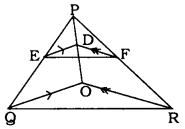Solution:
Data: In this figure. DE || OQ and DF || OR.
To Prove: EF || QR
Solution: In ∆POQ, DE || OQ.
$$\quad \frac{\mathrm{PE}}{\mathrm{EQ}}=\frac{\mathrm{PD}}{\mathrm{DO}} \quad \ldots \ldots \text { (i) }(\text { Theroem } 1)$$
Similarly. In ∆POR. DF || OR.
$$\quad \frac{\mathrm{PD}}{\mathrm{DO}}=\frac{\mathrm{PF}}{\mathrm{FR}} \quad \ldots \ldots \text { (ii) }(\text { Theorem } 1)$$
from equation (1) and (11), we have
$$\frac{P F}{E Q}=\frac{P D}{D O}=\frac{P F}{F R}$$
$$\frac{\mathrm{PE}}{\mathrm{EQ}}=\frac{\mathrm{PF}}{\mathrm{FR}}$$
In ∆PQR. if $$\frac{P E}{E Q}=\frac{P F}{F R}$$ then EF || QK. (∵ Theorem 1).

Question 6.
In the following figure, A, B an C are points on OP. OQ and OR respectively such that AB || PQ and AC || PR. Show that BC || QR.Solution:
Data: In ∆QPR, AB || PQ and AC || PR. A, B, and C are the points on OP. OQ and OR.
To Prove: BC || QR
Solution: In ∆OPQ, AB || PQ.
$$\quad \frac{\mathrm{OA}}{\mathrm{AP}}=\frac{\mathrm{OB}}{\mathrm{BQ}} \quad \ldots \ldots \text { (i) }(\text { Theorem } 1)$$
Similarly, In ∆OPR. AC|| PR.
$$\quad \frac{\mathrm{OA}}{\mathrm{AP}}=\frac{\mathrm{OC}}{\mathrm{CR}} \quad \ldots \ldots \text { (i) }(\text { Theorem } 1)$$
from equation (i) and (ii), we have
$$\frac{\mathrm{OA}}{\mathrm{AP}}=\frac{\mathrm{OB}}{\mathrm{BQ}}=\frac{\mathrm{OC}}{\mathrm{CR}}$$
$$\quad \frac{\mathrm{OB}}{\mathrm{BQ}}=\frac{\mathrm{OC}}{\mathrm{CR}}$$
∴ BC || QR (∵ Theorem 2)

Question 7.
Using Theorem 2.1, prove that a line drawn through the mid-point of one side of a triangle parallel to another side bisects the third side. (Recall that you have proved it in Class IX).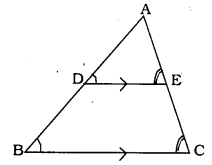Solution:
Data: In ∆ABC. D is the mid-point of AB.
DE Is drawn parallel to BC from D.
To Prove: DE bisects AC side at E.
∠D = ∠B (corresponding angles)
∠E = ∠C (corresponding angles)
$$\quad \frac{\mathrm{AD}}{\mathrm{AB}}=\frac{\mathrm{AE}}{\mathrm{AC}}$$
$$\frac{1}{2}=\frac{\mathrm{AE}}{\mathrm{AC}}$$
∴ AC = AE + EC
∴ E is the mid-point of AC
∴ DE bisects AC at E.

Question 8.
Using Theorem 2.2, prove that the line joining the mid-points of any two sides of a triangle Is parallel to the third side. (Recall that you have done it in Class IX)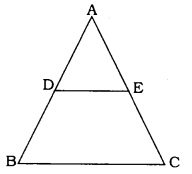Solution:
Solution: In ∆ABC, D and E are mid-points of AB and AC.
AE = EC
AC = 2AE
$$\frac{\mathrm{AD}}{\mathrm{AB}}=\frac{\mathrm{AE}}{\mathrm{AC}}=\frac{1}{2}$$
As per S.S.S. Postulate.
∴ They are equiangular triangles.
∴ ∠A is common.
These are pair of corresponding angles
∴ DE || BC

Question 9.
ABCD is a trapezium in which AB || DC and its diagonals intersect each other at the point O. Show that $$\frac{\mathrm{AO}}{\mathrm{CO}}=\frac{\mathrm{CO}}{\mathrm{DO}}$$
Solution:
Data : ABCD is a trapezium in which AB || DC and its diagonals intersect each other at the point O.
To Prove: $$\frac{\mathrm{AO}}{\mathrm{CO}}=\frac{\mathrm{CO}}{\mathrm{DO}}$$
Proof: In Trapezium ABCD. AB || DC.
∴ In ∆AOB and ∆DOC,
∠OCO = ∠OAB (alternate angles)∠ODC = ∠OBA (alternate angles)
∠DOC = ∠AOB (vertically opposite angles)
∴ ∆AOB and ∆DOC are equiangular triangles.
∴ ∆AOB ||| ∆DOC
Similar triangles divides sides in ratio.
$$\frac{\mathrm{AO}}{\mathrm{CO}}=\frac{\mathrm{CO}}{\mathrm{DO}}$$

Question 10.
The diagonals of a quadrilateral ABCD intersect each other at the point O such that $$\frac{\mathrm{AO}}{\mathrm{BO}}=\frac{\mathrm{CO}}{\mathrm{DO}}$$ . Show that ABCD is a trapezium.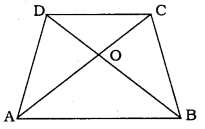Solution:
Data: In the quadrilateral ABCD. the diagonals intersect at 0’ such that $$\frac{\mathrm{AO}}{\mathrm{BO}}=\frac{\mathrm{CO}}{\mathrm{DO}}$$
To Prove: ABCD is a trapezium.
Solution: In the qudrilateral ABCD, $$\frac{\mathrm{AO}}{\mathrm{BO}}=\frac{\mathrm{CO}}{\mathrm{DO}}$$
$$\quad \frac{\mathrm{AO}}{\mathrm{CO}}=\frac{\mathrm{OB}}{\mathrm{OD}}$$
It means sides of ∆AOB and ∆DOC divides proportionately.
∴ ∆AOB ||| ∆DOC.
Similarly. ∆AOD ||| ∆BOC.
Now, ∆AOB + ∆AOD = ∆BOC + ∆DOC
∆ABD = ∆ABC.
Both triangles are on the same base AB and between two pair of lines and equal in area.
∴ AB || DC.

We hope the given KSEEB SSLC Class 10 Maths Solutions Chapter 2 Triangle Ex 2.2 will help you. If you have any query regarding Karnataka SSLC Class 10 Maths Solutions Chapter 2 Triangle Exercise 2.2, drop a comment below and we will get back to you at the earliest.

error: Content is protected !!